﻿ 基于TOF模式的UWB定位原理与精度验证文章快速检索 高级检索
 大地测量与地球动力学2020, Vol. 40Issue (3): 247-251  DOI: 10.14075/j.jgg.2020.03.006### 引用本文YANG Sheng, HU Zhigang, ZHAO Qile, et al. Principle and Precision Verification of UWB Positioning Based on TOF[J]. Journal of Geodesy and Geodynamics, 2020, 40(3): 247-251.### Corresponding author

HU Zhigang, associate processor, majors in GNSS, E-mail:frank27090201@163.com.

### 第一作者简介

YANG Sheng, postgraduate, majors in indoor positioning, E-mail:shyangwhu@163.com.

### 文章历史

1. 武汉大学卫星导航定位技术研究中心, 武汉市珞喻路129号, 430071

1 UWB/TOF测距原理

 $\mathrm{TOF}=\frac{1}{2} \times\left(\left(T_{3}-T_{0}\right)-\left(T_{2}-T_{1}\right)\right)$ (1)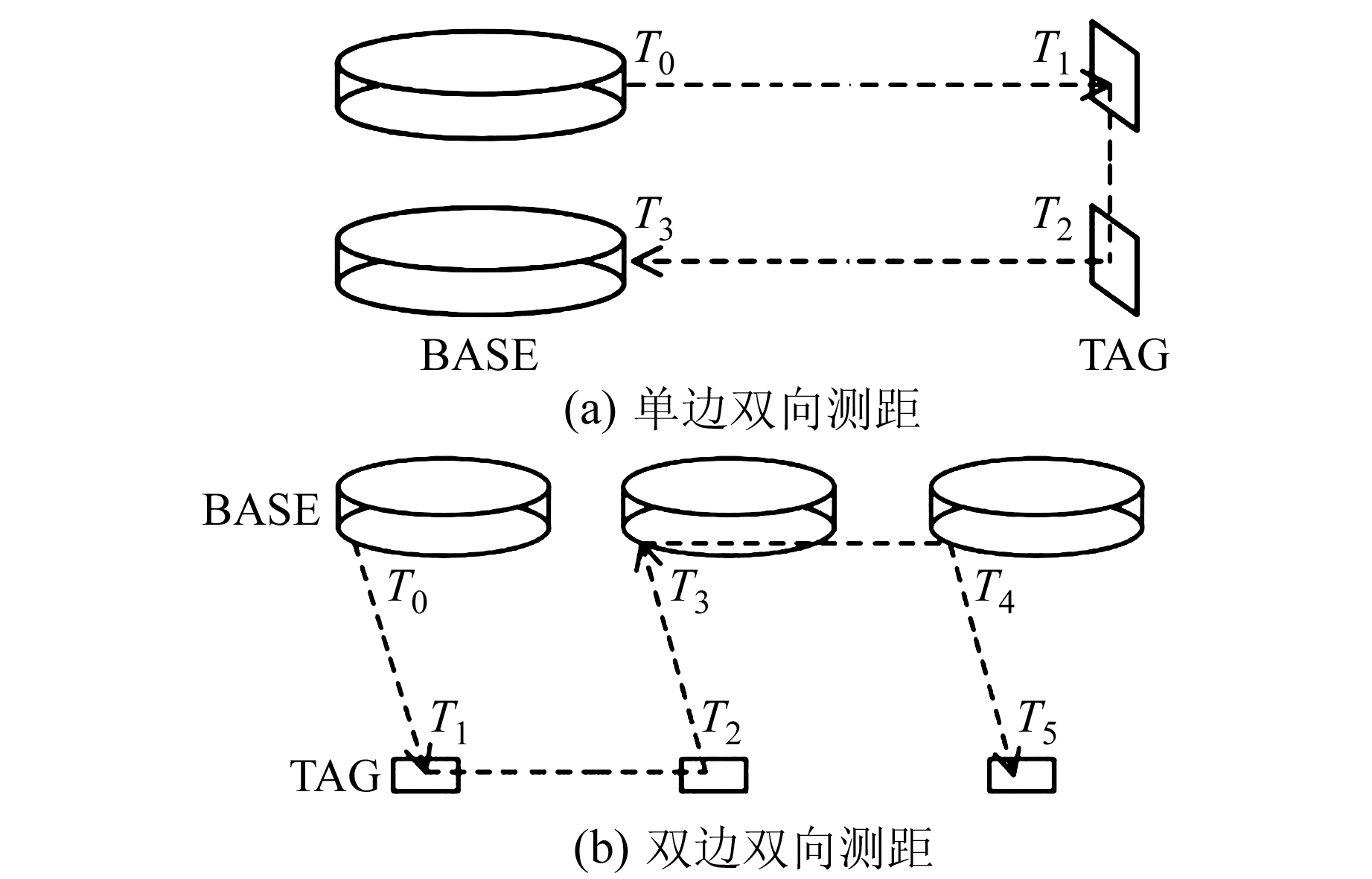图 1 两种TOF测距模式 Fig. 1 Two TOF measuring modes

 $|r|=\frac{1}{2} \times\left|k_{b}-k_{t}\right| \times T_{d} \times c$ (2)

 $\left\{ {\begin{array}{*{20}{l}} {{T_{r1}} = {T_3} - {T_0} \cdot {T_{p1}} = {T_2} - {T_1}}\\ {{T_{r2}} = {T_5} - {T_2}, {T_{p2}} = {T_1} - {T_3}}\\ {{\mathop{\rm TOF}\nolimits} = \frac{{{T_1} \times {T_2} - {T_{p1}} \times {T_{p2}}}}{{{T_{r1}} + {T_{r2}} + {T_{p1}} + {T_{p2}}}}} \end{array}} \right.$ (3)

 $|r| \approx \operatorname{TOF} \times \frac{\left|k_{b}+k_{t}\right|}{2} \times c$ (4)

kbkt均为20 ppm，若基站与标签距离为200 m，TOF约为666 ns，测距误差为：

 $|r|=666 \times 10^{-9} \times 20 \times 10^{-6} \times \\ 3 \times 10^{8} \approx 0.004 \mathrm{m}$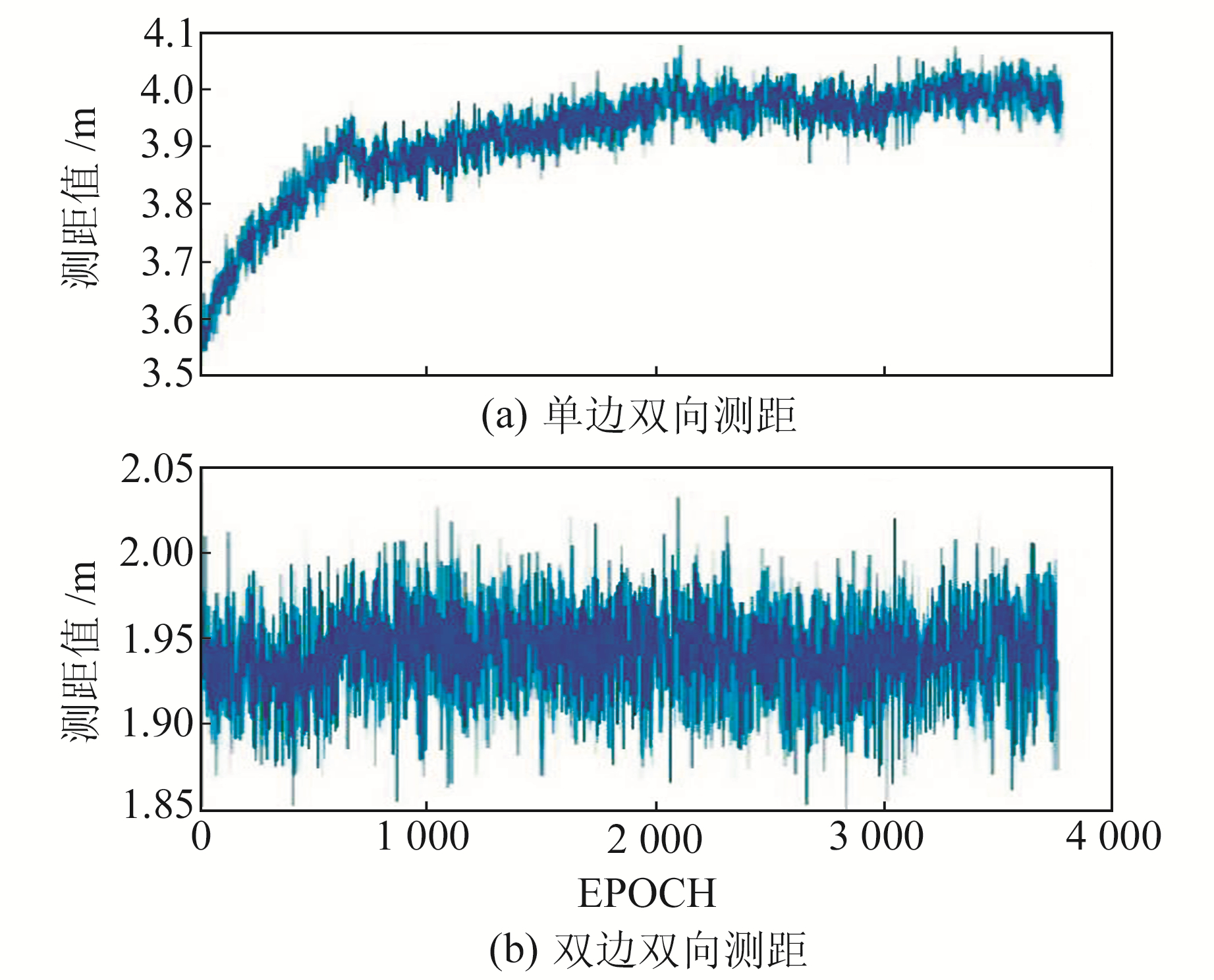图 2 单边双向测距与双边双向测距比较 Fig. 2 Comparison between SS-TWR and DS-TWR
2 TOF测距误差源分析 2.1 TOF测距粗差的剔除与降噪

 $\boldsymbol{X}_{k}={\mathit{\boldsymbol{ \boldsymbol{\varPhi} }}} \boldsymbol{X}_{k-1}+\boldsymbol{w}_{k}$ (5)

 ${\mathit{\boldsymbol{Z}}_k} = \mathit{\boldsymbol{H}}{\mathit{\boldsymbol{X}}_k} + {\mathit{\boldsymbol{v}}_k}$ (6)

 $\boldsymbol{K}_{k}=\boldsymbol{P}_{k, k-1} \boldsymbol{H}^{\mathrm{T}}\left(\boldsymbol{H} \boldsymbol{P}_{k, k-1} \boldsymbol{H}^{\mathrm{T}}+\boldsymbol{R}_{k}\right)^{-1}$ (7)

k时刻观测噪声Rk增大，Kk会相应减小，新观测值对系统状态和状态协方差矩阵的影响也相应减小，为此一个可行的粗差剔除思路为

1) 一步预测Xk, k-1Pk, k-1，并计算一步预测残差值rk= Zk- HXk, k-1

2) 计算rk的协方差矩阵Drk= HPk, k-1HT，检验量Δrk= rkTDrk-1rk

3) 对于给定阈值C，当Δrk < C，认为新观测值不包含粗差，否则认为新观测值包含粗差，修正观测值协方差矩阵$\boldsymbol{R}_{k}=\frac{\Delta \boldsymbol{r}_{{k}}}{C} \boldsymbol{R}_{k}$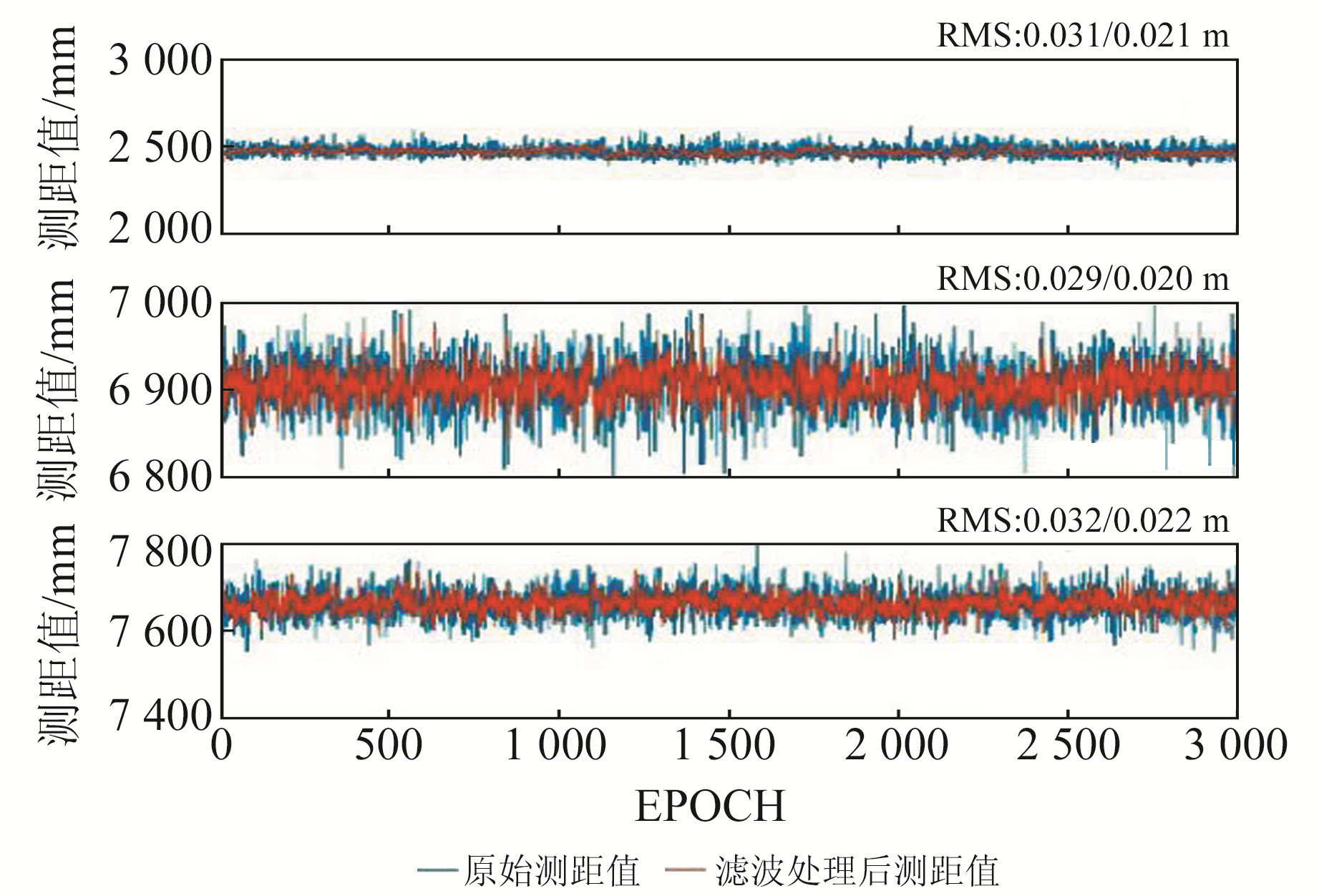图 3 卡尔曼滤波降噪效果 Fig. 3 Noise reduction effects of Kalman filter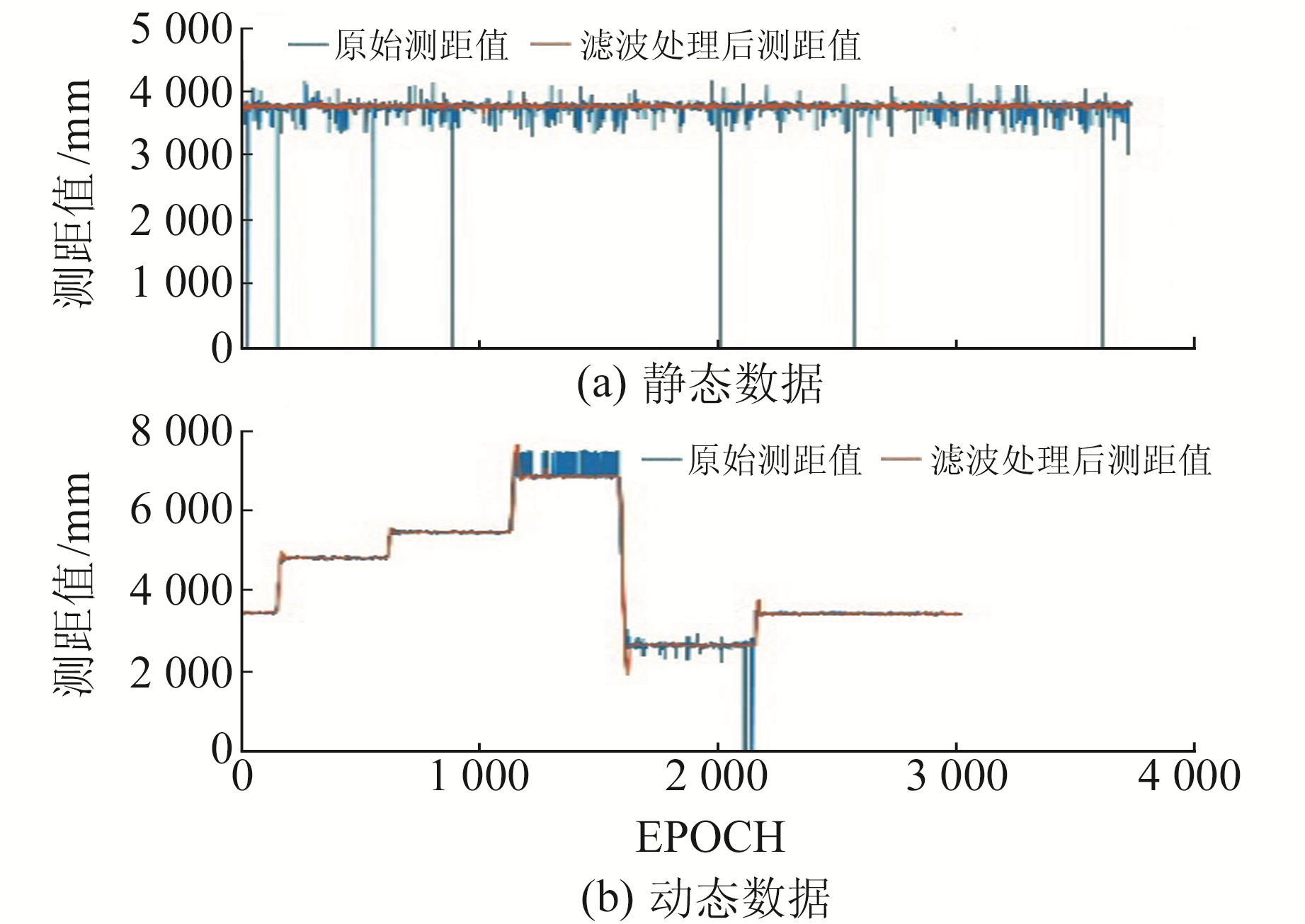图 4 卡尔曼滤波抗粗差效果 Fig. 4 Anti-bias effects of Kalman filter
2.2 多径效应与NLOS环境对TOF测距的影响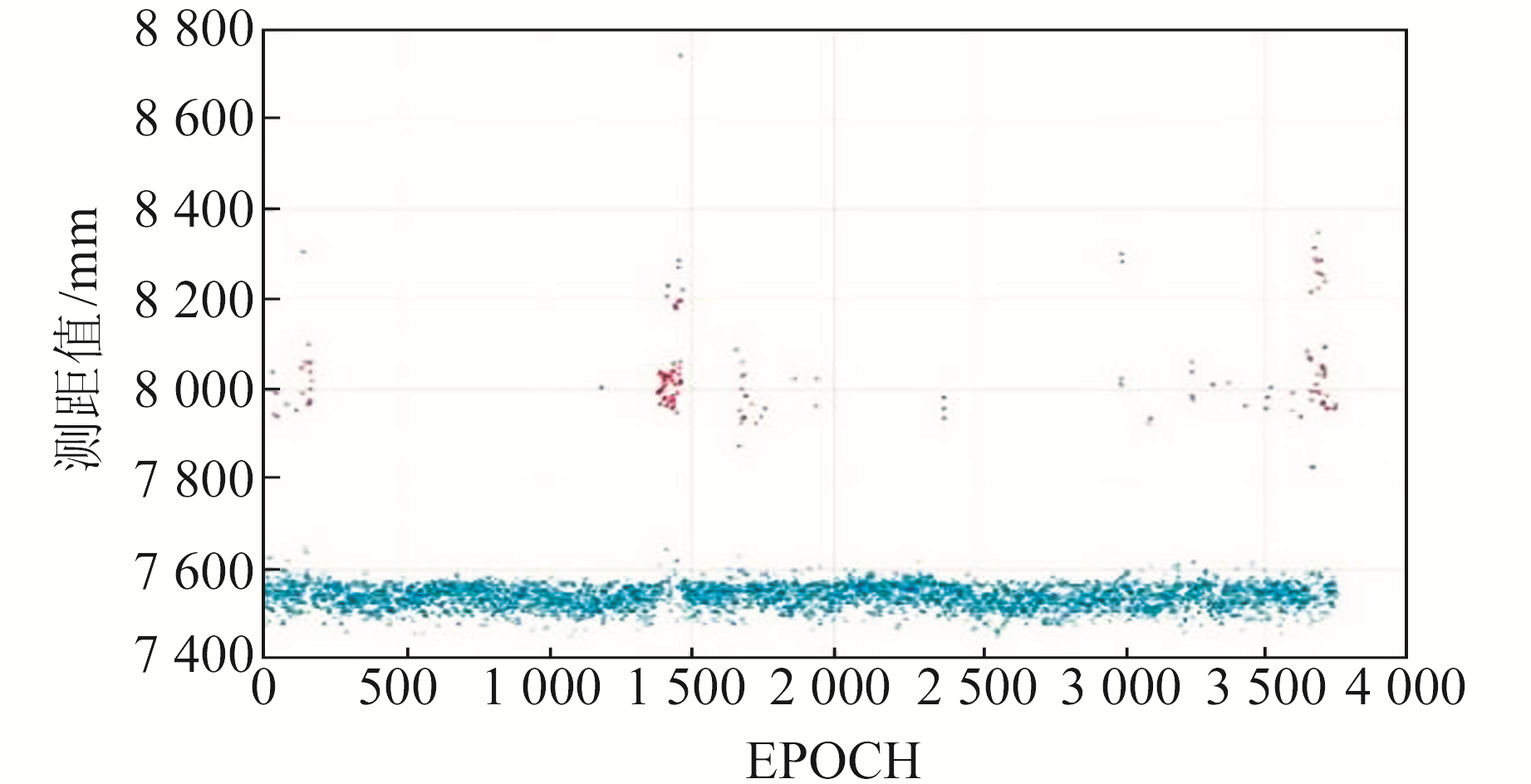图 5 多径效应对TOF测距的影响 Fig. 5 Multi-path effects on TOF measuring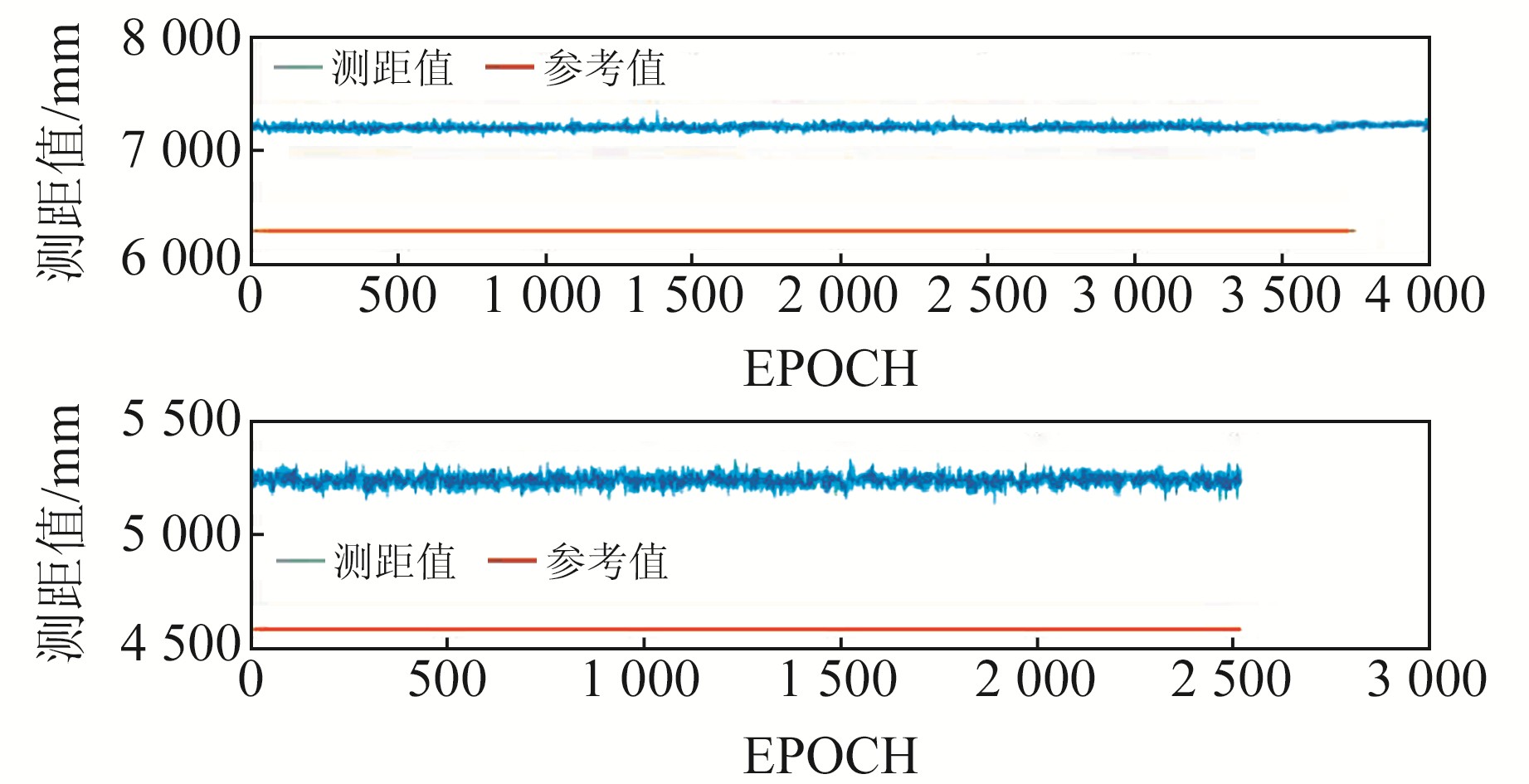图 6 NLOS环境下观测值与真值对比 Fig. 6 Observed and true value under NLOS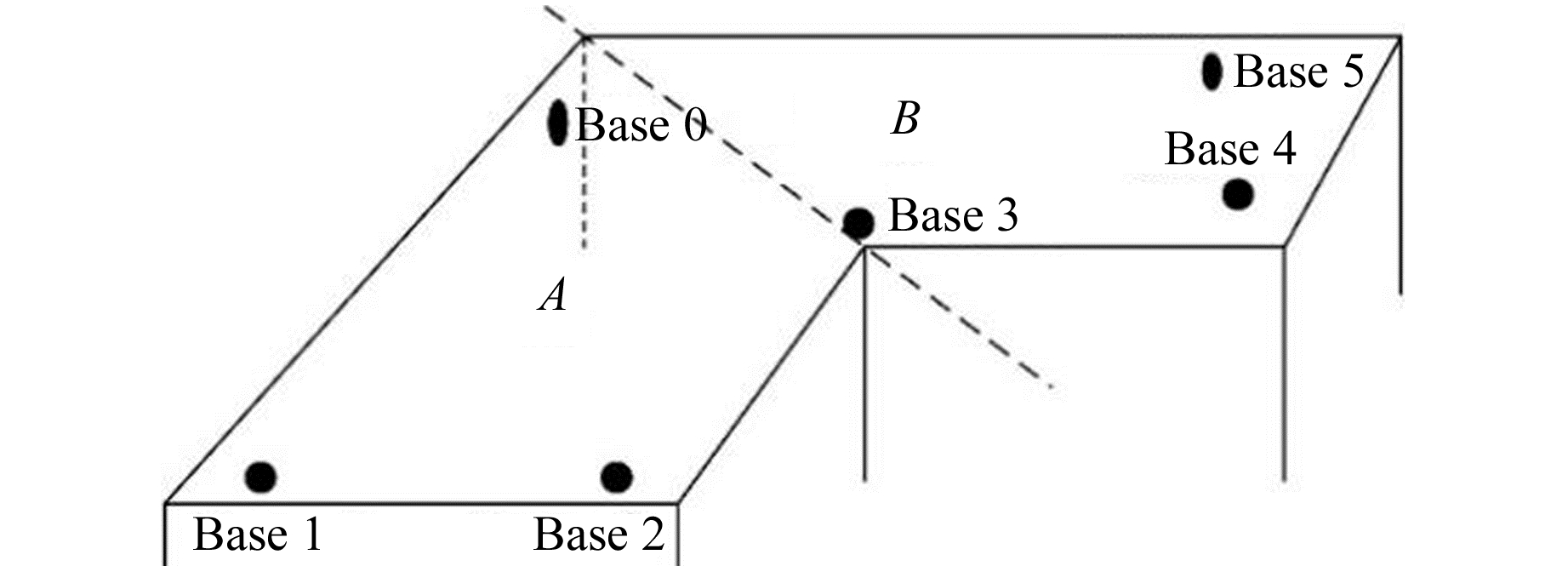图 7 “L”型空间及划分 Fig. 7 "L" shaped space and partitioning

1) 在空间内布设6个基站Base 0~Base 5；

2) 以虚线为界将空间划分成A和B 2个子块，标签位于A中时，只用Base 0、1、2、3定位，位于B中时只用Base 0、3、4、5定位；

3) 利用所有基站测距值初始化标签位置，判断标签位于哪一子块内并选择相应基站；

4) 标签所在子块发生变化时，更换相应基站。

3 定位模型及精度验证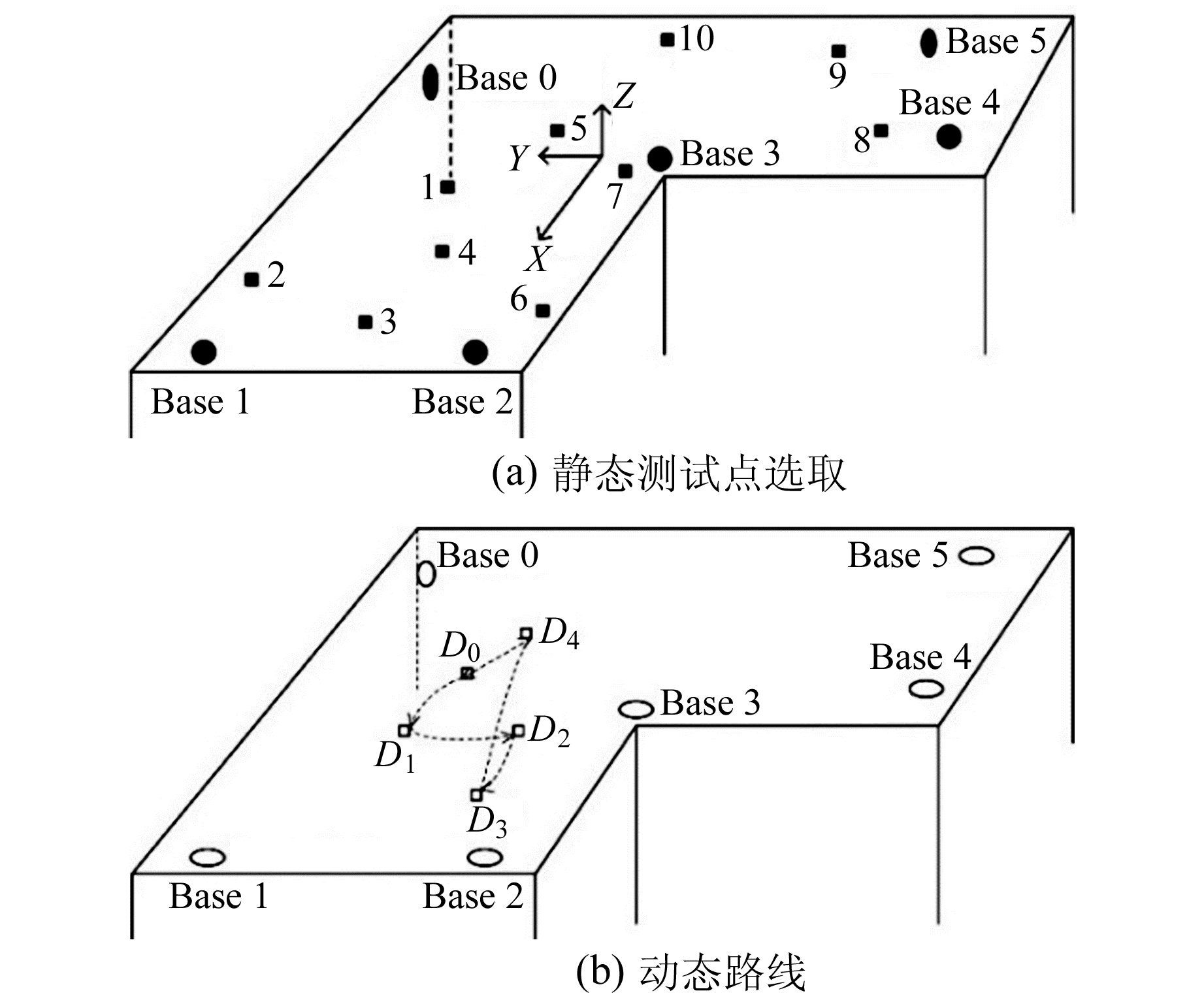图 8 基站布置与测试点选取 Fig. 8 Layout of base stations and selection of test points
3.1 定位算法

 $\boldsymbol{X}_{k}={\mathit{\boldsymbol{ \boldsymbol{\varPhi} }}} \boldsymbol{X}_{k-1}+\mathit{\boldsymbol{ \boldsymbol{\varGamma} }} \boldsymbol{w}_{k}$ (8)

 ${\mathit{\boldsymbol{ \boldsymbol{\varPhi} }}}_{6 \times 6}=\left[\begin{array}{cc}\boldsymbol{I}_{3 \times 3} & \boldsymbol{D} T \\ 0 & \boldsymbol{I}_{3 \times 3}\end{array}\right], \mathit{\boldsymbol{ \boldsymbol{\varGamma} }}_{6 \times 3}=\left[\begin{array}{c}0 \\ \boldsymbol{D} T\end{array}\right]$

 $\boldsymbol{Z}_{k}=f\left(\boldsymbol{X}_{k}\right)+\boldsymbol{v}_{k}$

 $d_{0}=\sqrt{\left(x_{0}-x\right)^{2}+\left(y_{0}-y\right)^{2}+\left(h_{0}-h\right)^{2}}+v_{0}$ (9)

 $d_{0}^{2}=x^{2}+y^{2}+h^{2}-2 x_{0} x-2 y_{0} y-2 h_{0} h+l_{0}^{2}$ (10)

 $\begin{array}{c} -2\left(x_{i}-x_{0}\right) x-2\left(y_{i}-y_{0}\right) y- \\ 2\left(h_{i}-h_{0}\right) h=d_{i}^{2}-d_{0}^{2}-\left(l_{i}^{2}-l_{0}^{2}\right) \end{array}$ (11)

 $h=\frac{1}{n} \sum\limits_{i=1}^{n}\left(h_{i}-\sqrt{d_{i}^{2}-x^{2}-y^{2}}\right)$ (12)

3.2 定位精度验证表 1 静态定位测试点误差 Tab. 1 Error of static positioning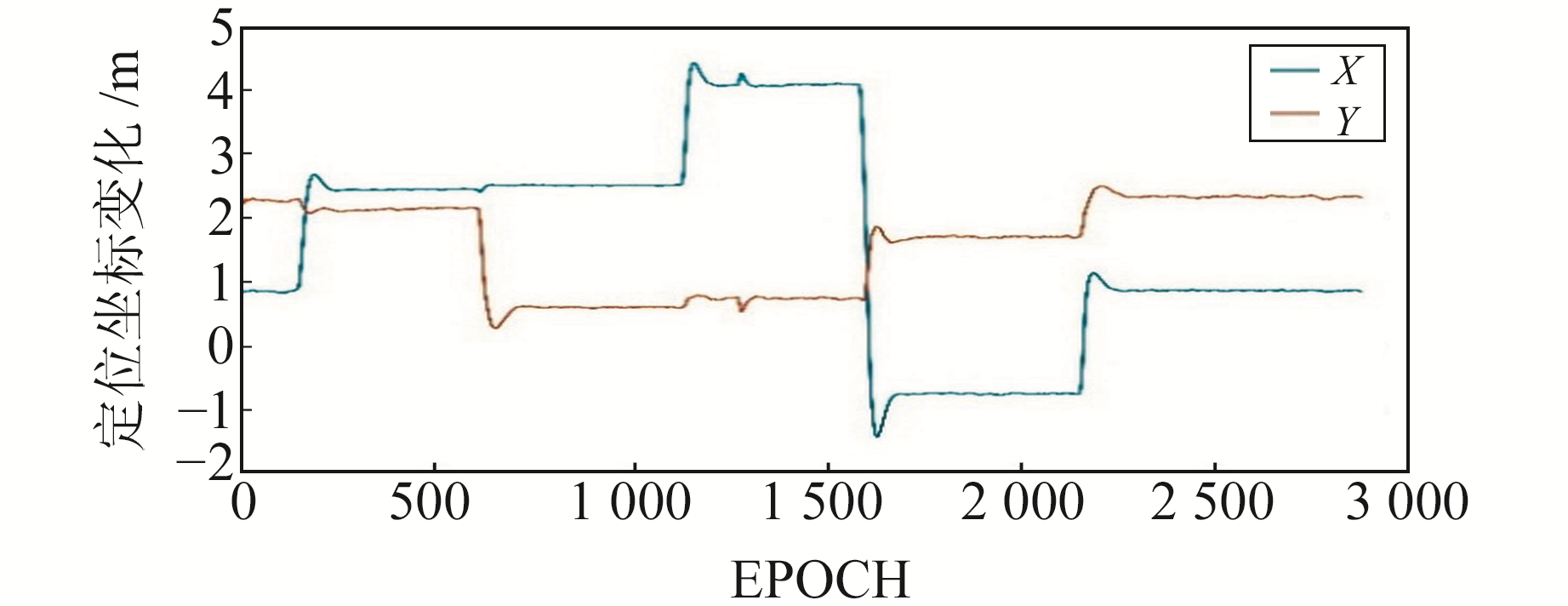图 9 动态定位水平坐标变化 Fig. 9 Changes of horizontal location in dynamic positioning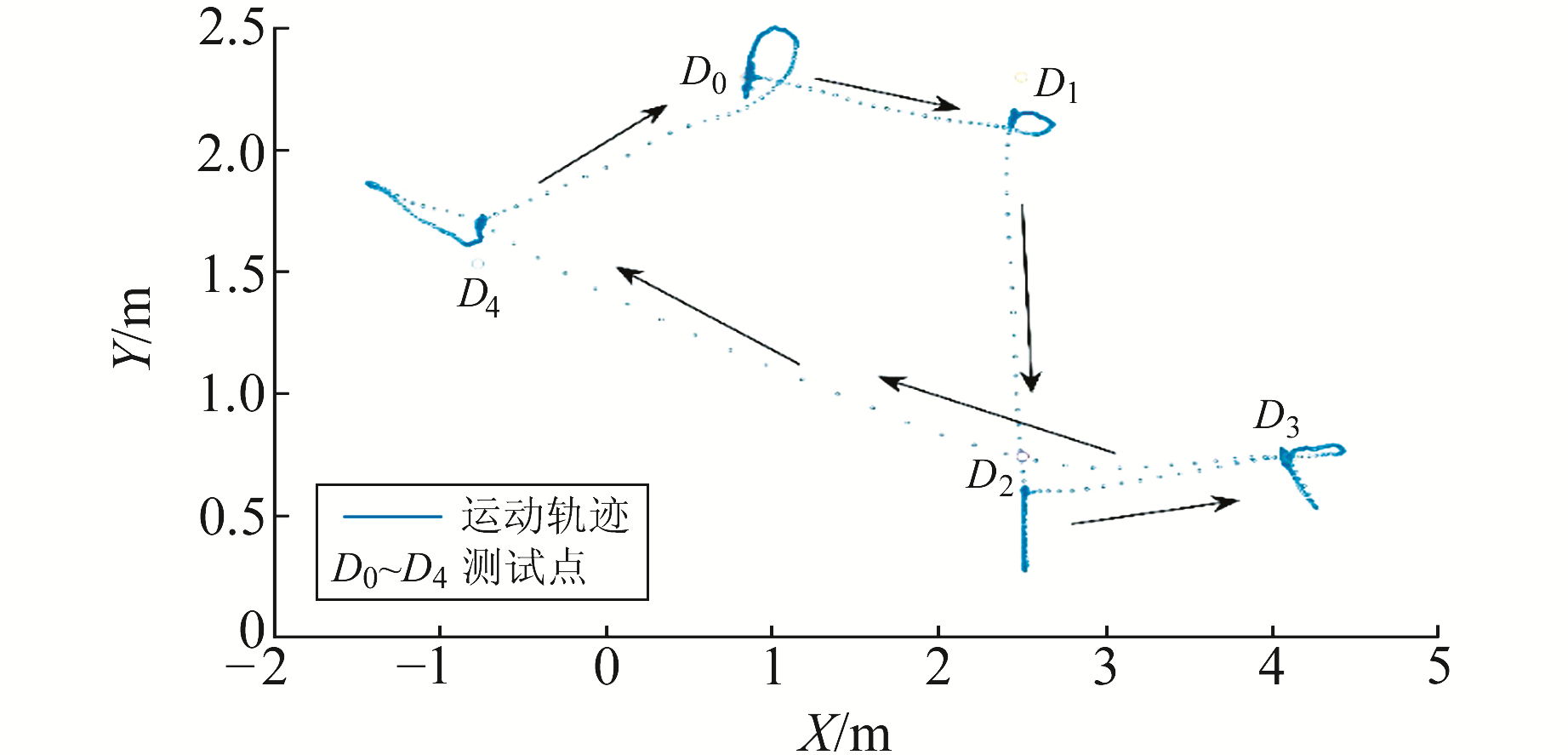图 10 平面动态轨迹 Fig. 10 Horizontal motion trail表 2 动态定位测试点误差 Tab. 2 Error of dynamic positioning
4 结语

1) 原始TOF测距经过滤波处理后，测距粗差能得到有效的剔除，测距值RMS由原始测量值的约3 cm提升至约2 cm。

2) 静态定位结果中，受多径效应与NLOS环境影响严重的区域水平精度降至约0.4 m，其他区域水平精度基本优于10 cm。

3) 对于动态定位，由于选取的区域通视条件较好且多径效应干扰较弱，滤波稳定后的水平精度优于0.2 m，部分点位水平精度可达10 cm左右。

  Alarifi A, Al-Salman A, Alsaleh M, et al. Ultra Wideband Indoor Positioning Technologies: Analysis and Recent Advances[J]. Sensors, 2016, 16(5): 1-36 DOI:10.1109/JSEN.2015.2509619 (0)  席瑞, 李玉军, 侯孟书. 室内定位方法综述[J]. 计算机科学, 2016, 43(4): 1-6 (Xi Rui, Li Yujun, Hou Mengshu. Survey on Indoor Locationing[J]. Computer Science, 2016, 43(4): 1-6) (0)  Chen L, Pei L, Kuusniemi H, et al. Bayesian Fusion for Indoor Positioning Using Bluetooth Fingerprints[J]. Wireless Personal Communications, 2013, 70(4): 1735-1745 DOI:10.1007/s11277-012-0777-1 (0)  陈国良, 张言哲, 汪云甲, 等. WiFi-PDR室内组合定位的无迹卡尔曼滤波算法[J]. 测绘学报, 2015, 44(12): 1314-1321 (Chen Guoliang, Zhang Yanzhe, Wang Yunjia, et al. Unscented Kalman Filter Algorithm for WiFi-PDR Integrated Indoor Positioning[J]. Acta Geodaetica et Cartographica Sinica, 2015, 44(12): 1314-1321 DOI:10.11947/j.AGCS.2015.20140691) (0)  牛群峰, 曹一帆, 王莉, 等. 基于TW-TOF的UWB室内定位技术与优化算法研究[J]. 自动化与仪表, 2018, 33(1): 5-9 (Niu Qunfeng, Cao Yifan, Wang Li, et al. Study of UWB Indoor Positioning Technology and Optimization Algorithm Based on TW-TOF[J]. Automation and Instrumentation, 2018, 33(1): 5-9) (0)  Xu B, Sun G D, Yu R, et al. High-Accuracy TDOA-Based Localization without Time Synchronization[J]. IEEE Transactions on Parallel and Distributed Systems, 2013, 24(8): 1567-1576 DOI:10.1109/TPDS.2012.248 (0)  Feng C, Au W S A, Valaee S, et al. Received-Signal-Strength-Based Indoor Positioning Using Compressive Sensing[J]. IEEE Transactions on Mobile Computing, 2012, 11(12): 1983-1993 DOI:10.1109/TMC.2011.216 (0)  Guvenc I, Chong C C, Watanabe F. NLOS Identification and Mitigation for UWB Localization Systems[C]. Wireless Communications and Networking Conference, Hong Kong, 2007 (0)  刘韬, 徐爱功, 隋心. 基于自适应抗差卡尔曼滤波的UWB室内定位[J]. 传感技术学报, 2018, 31(4): 567-572 (Liu Tao, Xu Aigong, Sui Xin. Adaptive Robust Kalman Filtering for UWB Indoor Positioning[J]. Chinese Journal of Sensors and Actuators, 2018, 31(4): 567-572 DOI:10.3969/j.issn.1004-1699.2018.04.013) (0)
Principle and Precision Verification of UWB Positioning Based on TOF
YANG Sheng1HU Zhigang1ZHAO Qile1     ZHANG Huichao1
1. GNSS Research Center, Wuhan University, 129 Luoyu Road, Wuhan 430071, China
Abstract: In this paper, we analyze the feature of ultra-wide band (UWB) pseudo range, which is generated by method of time of flight (TOF). By applying Kalman filter and its transformation to the original TOF measures, we detect and remove measures with error as well as lower the noise of TOF measures. We build a model to correct system error of TOF measures by comparing it with known distance. The positioning result shows that as system error gets well corrected, the precision of UWB positioning reaches a level of 10 cm in static mode, and a level of 0.2 m in kinematic mode, while the path of object keeps smooth.
Key words: indoor positioning; UWB; TOF; error detection and elimination